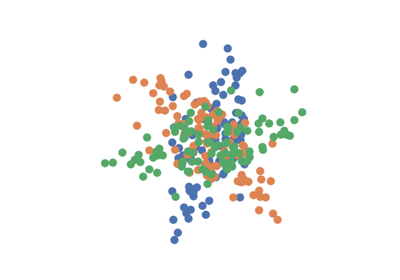Energy¶

class hyppo.ksample.Energy(compute_distance='euclidean', bias=False, **kwargs)

Energy test statistic and p-value.

Energy is a powerful multivariate 2-sample test. It leverages distance matrix capabilities (similar to tests like distance correlation or Dcorr). In fact, Energy statistic is equivalent to our 2-sample formulation nonparametric MANOVA via independence testing, i.e. hyppo.ksample.KSample, and to hyppo.independence.Dcorr, hyppo.ksample.DISCO, hyppo.independence.Hsic, and hyppo.ksample.MMD 1 2.

Parameters
• compute_distance (str, callable, or None, default: "euclidean") -- A function that computes the distance among the samples within each data matrix. Valid strings for compute_distance are, as defined in sklearn.metrics.pairwise_distances,

• From scikit-learn: ["euclidean", "cityblock", "cosine", "l1", "l2", "manhattan"] See the documentation for scipy.spatial.distance for details on these metrics.

• From scipy.spatial.distance: ["braycurtis", "canberra", "chebyshev", "correlation", "dice", "hamming", "jaccard", "kulsinski", "mahalanobis", "minkowski", "rogerstanimoto", "russellrao", "seuclidean", "sokalmichener", "sokalsneath", "sqeuclidean", "yule"] See the documentation for scipy.spatial.distance for details on these metrics.

Set to None or "precomputed" if x and y are already distance matrices. To call a custom function, either create the distance matrix before-hand or create a function of the form metric(x, **kwargs) where x is the data matrix for which pairwise distances are calculated and **kwargs are extra arguements to send to your custom function.

• bias (bool, default: False) -- Whether or not to use the biased or unbiased test statistics.

• **kwargs -- Arbitrary keyword arguments for compute_distance.

Notes

Traditionally, the formulation for the 2-sample Energy statistic is as follows 3:

Define $$\{ u_i \stackrel{iid}{\sim} F_U,\ i = 1, ..., n \}$$ and $$\{ v_j \stackrel{iid}{\sim} F_V,\ j = 1, ..., m \}$$ as two groups of samples deriving from different distributions with the same dimensionality. If $$d(\cdot, \cdot)$$ is a distance metric (i.e. Euclidean) then,

$\mathrm{Energy}_{n, m}(\mathbf{u}, \mathbf{v}) = \frac{1}{n^2 m^2} \left( 2nm \sum_{i = 1}^n \sum_{j = 1}^m d(u_i, v_j) - m^2 \sum_{i,j=1}^n d(u_i, u_j) - n^2 \sum_{i, j=1}^m d(v_i, v_j) \right)$

The implementation in the hyppo.ksample.KSample class (using hyppo.independence.Dcorr using 2 samples) is in fact equivalent to this implementation (for p-values) and statistics are equivalent up to a scaling factor 1.

The p-value returned is calculated using a permutation test uses hyppo.tools.perm_test. The fast version of the test uses hyppo.tools.chi2_approx.

References

1(1,2)

Sambit Panda, Cencheng Shen, Ronan Perry, Jelle Zorn, Antoine Lutz, Carey E. Priebe, and Joshua T. Vogelstein. Nonpar MANOVA via Independence Testing. arXiv:1910.08883 [cs, stat], April 2021. arXiv:1910.08883.

2

Cencheng Shen and Joshua T. Vogelstein. The exact equivalence of distance and kernel methods in hypothesis testing. AStA Advances in Statistical Analysis, September 2020. doi:10.1007/s10182-020-00378-1.

3

Gábor J. Székely and Maria L. Rizzo. Testing for equal distributions in high dimensions. InterStat, pages 2004.

Methods Summary

 Energy.statistic(x, y) Calulates the Energy test statistic. Energy.test(x, y[, reps, workers, auto, ...]) Calculates the Energy test statistic and p-value.

Energy.statistic(x, y)

Calulates the Energy test statistic.

Parameters

x,y (ndarray) -- Input data matrices. x and y must have the same number of dimensions. That is, the shapes must be (n, p) and (m, p) where n is the number of samples and p and q are the number of dimensions.

Returns

stat (float) -- The computed Energy statistic.

Energy.test(x, y, reps=1000, workers=1, auto=True, random_state=None)

Calculates the Energy test statistic and p-value.

Parameters
• x,y (ndarray) -- Input data matrices. x and y must have the same number of dimensions. That is, the shapes must be (n, p) and (m, p) where n is the number of samples and p and q are the number of dimensions.

• reps (int, default: 1000) -- The number of replications used to estimate the null distribution when using the permutation test used to calculate the p-value.

• workers (int, default: 1) -- The number of cores to parallelize the p-value computation over. Supply -1 to use all cores available to the Process.

• auto (bool, default: True) -- Automatically uses fast approximation when n and size of array is greater than 20. If True, and sample size is greater than 20, then hyppo.tools.chi2_approx will be run. Parameters reps and workers are irrelevant in this case. Otherwise, hyppo.tools.perm_test will be run.

Returns

• stat (float) -- The computed Energy statistic.

• pvalue (float) -- The computed Energy p-value.

Examples

>>> import numpy as np
>>> from hyppo.ksample import Energy
>>> x = np.arange(7)
>>> y = x
>>> stat, pvalue = Energy().test(x, y)
>>> '%.3f, %.1f' % (stat, pvalue)
'0.267, 1.0'

Examples using hyppo.ksample.Energy¶